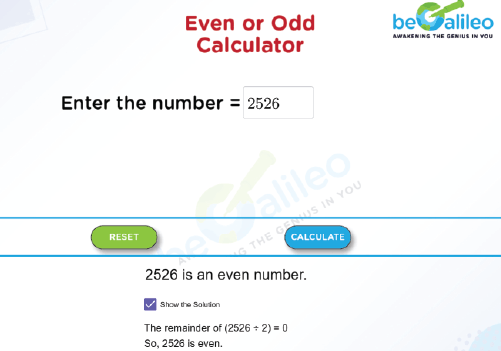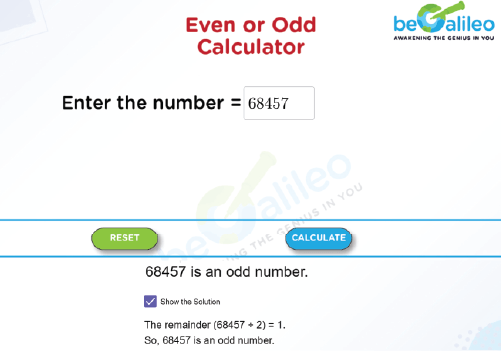>### Master Math with 1:1 Online Classes. Learn from Experts!# Even or odd calculator

An even or odd calculator is a simple and useful tool that determines whether a given number is even or odd.

Even numbers usually end in 0, 2, 4, 6, or 8. Each even number can be divided by 2. When divided by 2, even numbers leave no remainder.

Odd Numbers are those that end with 1, 3, 5, 7 or 9. Odd numbers can't be divided by 2 completely, leaving a remainder of 1.

## How to use the Even or Odd Calculator

Step 1 : Enter the number.

Step 2 : Click “CALCULATE” and then the answer will be displayed.

Step 3 : To see the steps involved in finding the solution, check the "Show the Solution"
box and the steps needed to solve the problem will be revealed.

Step 4 : Further, click the “RESET” button to enter the next number.

## How can we find whether a number is even or odd?

Any of the following techniques can be used to determine if a number is even or odd.

Method 1 :

• Look at the ones place of the number.
• If the number in the ones place is 0, 2, 4, 6, or 8, the given number is even.
• If the number in the ones place is 1, 3, 5, 7 or 9, then the given number is odd.

Method 2 :

• Divide the number by 2.
• If the Remainder is 0, then the number is even.
• If the Remainder is 1, then the number is odd.

## Solved examples

### Find whether the given numbers are odd or even. Q1. 2526

Solution :
The number in the ones place is 6.
2526\divide2 = 1236 R 0
So, the remainder of (2526\divide2) = 0
Therefore, 2526 is even.### Q2. 68457

Solution :
The number in the ones place is 7.
68457 \divide2 = 34228 R 1
So, the remainder of (68457\divide2) = 1
Therefore, 68457 is odd.